Calculus Volume 3

# Chapter 5

### Checkpoint

5.1

$V = ∑ i = 1 2 ∑ j = 1 2 f ( x i j * , y i j * ) Δ A = 0 V = ∑ i = 1 2 ∑ j = 1 2 f ( x i j * , y i j * ) Δ A = 0$

5.2

a. 26 b. Answers may vary.

5.3

$− 1340 3 − 1340 3$

5.4

$4 − ln 5 ln 5 4 − ln 5 ln 5$

5.5

$π 2 π 2$

5.6

Answers to both parts a. and b. may vary.

5.7

Type I and Type II are expressed as ${(x,y)|0≤x≤2,x2≤y≤2x}{(x,y)|0≤x≤2,x2≤y≤2x}$ and ${(x,y)|0≤y≤4,12y≤x≤y},{(x,y)|0≤y≤4,12y≤x≤y},$ respectively.

5.8

$π / 4 π / 4$

5.9

${ ( x , y ) | 0 ≤ y ≤ ln 2 , 1 ≤ x ≤ e y } ∪ { ( x , y ) | ln 2 ≤ y ≤ e , 1 ≤ x ≤ 2 } ∪ { ( x , y ) | e ≤ y ≤ e 2 , ln y ≤ x ≤ 2 } { ( x , y ) | 0 ≤ y ≤ ln 2 , 1 ≤ x ≤ e y } ∪ { ( x , y ) | ln 2 ≤ y ≤ e , 1 ≤ x ≤ 2 } ∪ { ( x , y ) | e ≤ y ≤ e 2 , ln y ≤ x ≤ 2 }$

5.10

Same as in the example shown.

5.11

$216 35 216 35$

5.12

$e24+10e−494e24+10e−494$ cubic units

5.13

$814814$ square units

5.14

$3 4 3 4$

5.15

$π 4 π 4$

5.16

$55 72 ≈ 0.7638 55 72 ≈ 0.7638$

5.17

$14 3 14 3$

5.18

$8 π 8 π$

5.19

$π/8π/8$

5.20

$V=∫02π∫022(16−2r2)rdrdθ=64πV=∫02π∫022(16−2r2)rdrdθ=64π$ cubic units

5.21

$A = 2 ∫ − π / 2 π / 6 ∫ 1 + sin θ 3 − 3 sin θ r d r d θ = 8 π + 9 3 A = 2 ∫ − π / 2 π / 6 ∫ 1 + sin θ 3 − 3 sin θ r d r d θ = 8 π + 9 3$

5.22

$π4π4$

5.23

$∭ B z sin x cos y d V = 8 ∭ B z sin x cos y d V = 8$

5.24

$∭ E 1 d V = ∫ x = −3 x = 3 ∫ y = − 9 − x 2 y = 9 − x 2 ∫ z = − 9 − x 2 − y 2 z = 9 − x 2 − y 2 1 d z d y d x = 36 π . ∭ E 1 d V = ∫ x = −3 x = 3 ∫ y = − 9 − x 2 y = 9 − x 2 ∫ z = − 9 − x 2 − y 2 z = 9 − x 2 − y 2 1 d z d y d x = 36 π .$

5.25

(i) $∫z=0z=4∫x=0x=4−z∫y=x2y=4−zf(x,y,z)dydxdz,∫z=0z=4∫x=0x=4−z∫y=x2y=4−zf(x,y,z)dydxdz,$ (ii) $∫y=0y=4∫z=0z=4−y∫x=0x=yf(x,y,z)dxdzdy,∫y=0y=4∫z=0z=4−y∫x=0x=yf(x,y,z)dxdzdy,$ (iii) $∫y=0y=4∫x=0x=y∫z=0z=4−yf(x,y,z)dzdxdy,∫y=0y=4∫x=0x=y∫z=0z=4−yf(x,y,z)dzdxdy,$ (iv) $∫x=0x=2∫y=x2y=4∫z=0z=4−yf(x,y,z)dzdydx,∫x=0x=2∫y=x2y=4∫z=0z=4−yf(x,y,z)dzdydx,$ (v) $∫x=0x=2∫z=0z=4−x2∫y=x2y=4−zf(x,y,z)dydzdx∫x=0x=2∫z=0z=4−x2∫y=x2y=4−zf(x,y,z)dydzdx$

5.26

$f ave = 8 f ave = 8$

5.27

$8 8$

5.28

$∭ E f ( r , θ , z ) r d z d r d θ = ∫ θ = 0 θ = π ∫ r = 0 r = 2 sin θ ∫ z = 0 z = 4 − r sin θ f ( r , θ , z ) r d z d r d θ . ∭ E f ( r , θ , z ) r d z d r d θ = ∫ θ = 0 θ = π ∫ r = 0 r = 2 sin θ ∫ z = 0 z = 4 − r sin θ f ( r , θ , z ) r d z d r d θ .$

5.29

$E={(r,θ,z)|0≤θ≤2π,0≤z≤1,z≤r≤2−z2}E={(r,θ,z)|0≤θ≤2π,0≤z≤1,z≤r≤2−z2}$ and $V=∫r=0r=1∫z=rz=2−r2∫θ=0θ=2πrdθdzdr.V=∫r=0r=1∫z=rz=2−r2∫θ=0θ=2πrdθdzdr.$

5.30

$E2={(r,θ,z)|0≤θ≤2π,0≤r≤1,r≤z≤4−r2}E2={(r,θ,z)|0≤θ≤2π,0≤r≤1,r≤z≤4−r2}$ and $V=∫r=0r=1∫z=rz=4−r2∫θ=0θ=2πrdθdzdr.V=∫r=0r=1∫z=rz=4−r2∫θ=0θ=2πrdθdzdr.$

5.31

$V ( E ) = ∫ θ = 0 θ = 2 π ∫ ϕ = 0 φ = π / 3 ∫ ρ = 0 ρ = 2 ρ 2 sin φ d ρ d φ d θ V ( E ) = ∫ θ = 0 θ = 2 π ∫ ϕ = 0 φ = π / 3 ∫ ρ = 0 ρ = 2 ρ 2 sin φ d ρ d φ d θ$

5.32

Rectangular: $∫x=−2x=2∫y=−4−x2y=4−x2∫z=−4−x2−y2z=4−x2−y2dzdydx−∫x=−1x=1∫y=−1−x2y=1−x2∫z=−4−x2−y2z=4−x2−y2dzdydx.∫x=−2x=2∫y=−4−x2y=4−x2∫z=−4−x2−y2z=4−x2−y2dzdydx−∫x=−1x=1∫y=−1−x2y=1−x2∫z=−4−x2−y2z=4−x2−y2dzdydx.$
Cylindrical: $∫θ=0θ=2π∫r=1r=2∫z=−4−r2z=4−r2rdzdrdθ.∫θ=0θ=2π∫r=1r=2∫z=−4−r2z=4−r2rdzdrdθ.$
Spherical: $∫φ=π/6φ=5π/6∫θ=0θ=2π∫ρ=cscφρ=2ρ2sinφdρdθdφ.∫φ=π/6φ=5π/6∫θ=0θ=2π∫ρ=cscφρ=2ρ2sinφdρdθdφ.$

5.33

$9 π 8 kg 9 π 8 kg$

5.34

$Mx=81π64Mx=81π64$ and $My=81π64My=81π64$

5.35

$x−=Mym=81π/649π/8=98x−=Mym=81π/649π/8=98$ and $y−=Mxm=81π/649π/8=98.y−=Mxm=81π/649π/8=98.$

5.36

$x−=Mym=1/201/12=35x−=Mym=1/201/12=35$ and $y−=Mxm=1/241/12=12y−=Mxm=1/241/12=12$

5.37

$x c = M y m = 1 / 15 1 / 6 = 2 5 and y c = M x m = 1 / 12 1 / 6 = 1 2 x c = M y m = 1 / 15 1 / 6 = 2 5 and y c = M x m = 1 / 12 1 / 6 = 1 2$

5.38

$Ix=∫x=0x=2∫y=0y=xy2xydydx=6435Ix=∫x=0x=2∫y=0y=xy2xydydx=6435$ and $Iy=∫x=0x=2∫y=0y=xx2xydydx=6435.Iy=∫x=0x=2∫y=0y=xx2xydydx=6435.$ Also, $I0=∫x=0x=2∫y=0y=x(x2+y2)xydydx=12835.I0=∫x=0x=2∫y=0y=x(x2+y2)xydydx=12835.$

5.39

$Rx=63535,Rx=63535,$ $Ry=63535,Ry=63535,$ and $R0=67035.R0=67035.$

5.40

$54 35 = 1.543 54 35 = 1.543$

5.41

$( 3 2 , 9 8 , 1 2 ) ( 3 2 , 9 8 , 1 2 )$

5.42

The moments of inertia of the tetrahedron $QQ$ about the $yz-plane,yz-plane,$ the $xz-plane,xz-plane,$ and the $xy-planexy-plane$ are $99/35,36/7,and243/35,99/35,36/7,and243/35,$ respectively.

5.43

$T−1(x,y)=(u,v)T−1(x,y)=(u,v)$ where $u=3x−y3u=3x−y3$ and $v=y3v=y3$

5.44

$J ( u , v ) = ∂ ( x , y ) ∂ ( u , v ) = | ∂ x ∂ u ∂ x ∂ v ∂ y ∂ u ∂ y ∂ v | = | 1 1 0 2 | = 2 J ( u , v ) = ∂ ( x , y ) ∂ ( u , v ) = | ∂ x ∂ u ∂ x ∂ v ∂ y ∂ u ∂ y ∂ v | = | 1 1 0 2 | = 2$

5.45

$∫ 0 π / 2 ∫ 0 1 r 3 d r d θ ∫ 0 π / 2 ∫ 0 1 r 3 d r d θ$

5.46

$x=12(v+u)x=12(v+u)$ and $y=12(v−u)y=12(v−u)$ and $∫24∫−uu4u2(12)dvdu.∫24∫−uu4u2(12)dvdu.$

5.47

$1 2 ( sin 2 − 2 ) 1 2 ( sin 2 − 2 )$

5.48

$∫ 0 3 ∫ 0 2 ∫ 1 2 ( v 3 + v w 3 u ) d u d v d w = 2 + ln 8 ∫ 0 3 ∫ 0 2 ∫ 1 2 ( v 3 + v w 3 u ) d u d v d w = 2 + ln 8$

### Section 5.1 Exercises

1.

27.

3.

0.

5.

21.3.

7.

a. 28 $ft3ft3$ b. 1.75 ft.

9.

a. $0.1120.112$ b. $fave≃0.175;fave≃0.175;$ here $f(0.4,0.2)≃0.1,f(0.4,0.2)≃0.1,$ $f(0.2,0.6)≃−0.2,f(0.2,0.6)≃−0.2,$ $f(0.8,0.2)≃0.6,f(0.8,0.2)≃0.6,$ and $f(0.8,0.6)≃0.2.f(0.8,0.6)≃0.2.$

11.

$2 π . 2 π .$

13.

40.

15.

$81 2 + 39 2 3 . 81 2 + 39 2 3 .$

17.

$e − 1 . e − 1 .$

19.

$15 − 10 2 9 . 15 − 10 2 9 .$

21.

0.

23.

$( e − 1 ) ( 1 + sin 1 − cos 1 ) . ( e − 1 ) ( 1 + sin 1 − cos 1 ) .$

25.

$3 4 ln ( 5 3 ) + 2 ln 2 2 − ln 2 . 3 4 ln ( 5 3 ) + 2 ln 2 2 − ln 2 .$

27.

$1 8 [ ( 2 3 − 3 ) π + 6 ln 2 ] . 1 8 [ ( 2 3 − 3 ) π + 6 ln 2 ] .$

29.

$1 4 e 4 ( e 4 − 1 ) . 1 4 e 4 ( e 4 − 1 ) .$

31.

$4 ( e − 1 ) ( 2 − e ) . 4 ( e − 1 ) ( 2 − e ) .$

33.

$− π 4 + ln ( 5 4 ) − 1 2 ln 2 + arctan 2 . − π 4 + ln ( 5 4 ) − 1 2 ln 2 + arctan 2 .$

35.

$1 2 . 1 2 .$

37.

$1 2 ( 2 cosh 1 + cosh 2 − 3 ) . 1 2 ( 2 cosh 1 + cosh 2 − 3 ) .$

49.

a. $f(x,y)=12xy(x2+y2)f(x,y)=12xy(x2+y2)$ b. $V=∫01∫01f(x,y)dxdy=18V=∫01∫01f(x,y)dxdy=18$ c. $fave=18;fave=18;$
d.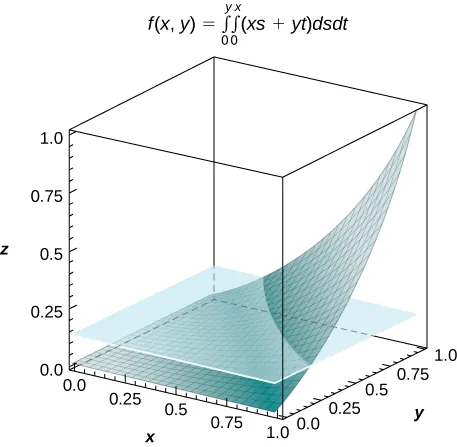53.

a. For $m=n=2,m=n=2,$ $I=4e−0.5≈2.43I=4e−0.5≈2.43$ b. $fave=e−0.5≃0.61;fave=e−0.5≃0.61;$
c.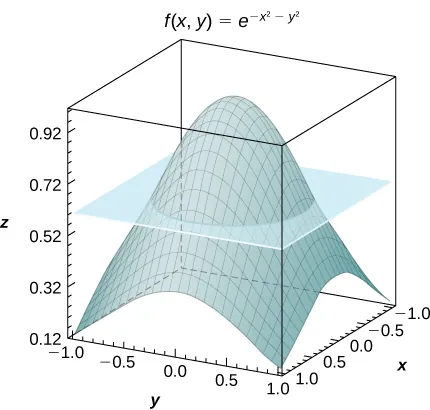55.

a. $2n+1+142n+1+14$ b. $1414$

59.

$56.5°56.5°$ F; here $f(x1*,y1*)=71,f(x1*,y1*)=71,$ $f(x2*,y1*)=72,f(x2*,y1*)=72,$ $f(x2*,y1*)=40,f(x2*,y1*)=40,$ $f(x2*,y2*)=43,f(x2*,y2*)=43,$ where $xi*xi*$ and $yj*yj*$ are the midpoints of the subintervals of the partitions of $[a,b][a,b]$ and $[c,d],[c,d],$ respectively.

### Section 5.2 Exercises

61.

$27 20 27 20$

63.

Type I but not Type II

65.

$π 2 π 2$

67.

$1 6 ( 8 + 3 π ) 1 6 ( 8 + 3 π )$

69.

$1000 3 1000 3$

71.

Type I and Type II

73.

The region $DD$ is not of Type I: it does not lie between two vertical lines and the graphs of two continuous functions $g1(x)g1(x)$ and $g2(x).g2(x).$ The region $DD$ is not of Type II: it does not lie between two horizontal lines and the graphs of two continuous functions $h1(y)h1(y)$ and $h2(y).h2(y).$

75.

$π 2 π 2$

77.

$0 0$

79.

$2 3 2 3$

81.

$41 20 41 20$

83.

$−63 −63$

85.

$π π$

87.

a. Answers may vary; b. $2323$

89.

a. Answers may vary; b. $7373$

91.

$8 π 3 8 π 3$

93.

$e − 3 2 e − 3 2$

95.

$1 3 1 3$

97.

$∫ 0 1 ∫ x − 1 1 − x x d y d x = ∫ −1 0 ∫ 0 y + 1 x d x d y + ∫ 0 1 ∫ 0 1 − y x d x d y = 1 3 ∫ 0 1 ∫ x − 1 1 − x x d y d x = ∫ −1 0 ∫ 0 y + 1 x d x d y + ∫ 0 1 ∫ 0 1 − y x d x d y = 1 3$

99.

$∫ −1 1 ∫ − 1 – y 2 1 – y 2 y d x d y = ∫ −1 1 ∫ − 1 – x 2 1 – x 2 y d y d x = 0 ∫ −1 1 ∫ − 1 – y 2 1 – y 2 y d x d y = ∫ −1 1 ∫ − 1 – x 2 1 – x 2 y d y d x = 0$

101.

$∬ D ( x 2 − y 2 ) d A = ∫ −1 1 ∫ y 4 − 1 1 − y 4 ( x 2 − y 2 ) d x d y = 464 4095 ∬ D ( x 2 − y 2 ) d A = ∫ −1 1 ∫ y 4 − 1 1 − y 4 ( x 2 − y 2 ) d x d y = 464 4095$

103.

$4 5 4 5$

105.

$5 π 32 5 π 32$

109.

$1 1$

111.

$2 2$

113.

a. $13;13;$ b. $16;16;$ c. $1616$

115.

a. $43;43;$ b. $2π;2π;$ c. $6π−436π−43$

117.

$0and0.865474;0and0.865474;$ $A(D)=0.621135A(D)=0.621135$

119.

$P[X+Y≤6]=1+32e2−5e6/5≈0.45;P[X+Y≤6]=1+32e2−5e6/5≈0.45;$ there is a $45%45%$ chance that a customer will spend $66$ minutes in the drive-thru line.

### Section 5.3 Exercises

123.

$D = { ( r , θ ) | 4 ≤ r ≤ 5 , π 2 ≤ θ ≤ π } D = { ( r , θ ) | 4 ≤ r ≤ 5 , π 2 ≤ θ ≤ π }$

125.

$D = { ( r , θ ) | 0 ≤ r ≤ 2 , 0 ≤ θ ≤ π } D = { ( r , θ ) | 0 ≤ r ≤ 2 , 0 ≤ θ ≤ π }$

127.

$D = { ( r , θ ) | 0 ≤ r ≤ 4 sin θ , 0 ≤ θ ≤ π } D = { ( r , θ ) | 0 ≤ r ≤ 4 sin θ , 0 ≤ θ ≤ π }$

129.

$D = { ( r , θ ) | 3 ≤ r ≤ 5 , π 4 ≤ θ ≤ π 2 } D = { ( r , θ ) | 3 ≤ r ≤ 5 , π 4 ≤ θ ≤ π 2 }$

131.

$D = { ( r , θ ) | 3 ≤ r ≤ 5 , 3 π 4 ≤ θ ≤ 5 π 4 } D = { ( r , θ ) | 3 ≤ r ≤ 5 , 3 π 4 ≤ θ ≤ 5 π 4 }$

133.

$D = { ( r , θ ) | 0 ≤ r ≤ tan θ sec θ , 0 ≤ θ ≤ π 4 } D = { ( r , θ ) | 0 ≤ r ≤ tan θ sec θ , 0 ≤ θ ≤ π 4 }$

135.

$0 0$

137.

$63 π 16 63 π 16$

139.

$3367 π 18 3367 π 18$

141.

$35 π 2 576 35 π 2 576$

143.

$7 288 π 2 [ 21 − e 2 + e 4 ] 7 288 π 2 [ 21 − e 2 + e 4 ]$

145.

$5 2 ln ( 1 + 2 ) 5 2 ln ( 1 + 2 )$

147.

$1 6 ( 2 − 2 ) 1 6 ( 2 − 2 )$

149.

$∫ 0 π ∫ 0 2 r 5 d r d θ = 32 π 3 ∫ 0 π ∫ 0 2 r 5 d r d θ = 32 π 3$

151.

$∫ − π / 2 π / 2 ∫ 0 4 r sin ( r 2 ) d r d θ = π sin 2 8 ∫ − π / 2 π / 2 ∫ 0 4 r sin ( r 2 ) d r d θ = π sin 2 8$

153.

$3 π 4 3 π 4$

155.

$π 2 π 2$

157.

$1 3 ( 4 π − 3 3 ) 1 3 ( 4 π − 3 3 )$

159.

$16 3 π 16 3 π$

161.

$π 18 π 18$

163.

a. $2π3;2π3;$ b. $π2;π2;$ c. $π6π6$

165.

$256 π 3 cm 3 256 π 3 cm 3$

167.

$3 π 32 3 π 32$

169.

$4 π 4 π$

171.

$π 4 π 4$

173.

$1 2 π e ( e − 1 ) 1 2 π e ( e − 1 )$

175.

$3 − π 4 3 − π 4$

177.

$133 864 π 3 133 864 π 3$

### Section 5.4 Exercises

181.

$192 192$

183.

$0 0$

185.

$∫ 1 2 ∫ 2 3 ∫ 0 1 ( x 2 + ln y + z ) d z d x d y = 35 6 + 2 ln 2 ∫ 1 2 ∫ 2 3 ∫ 0 1 ( x 2 + ln y + z ) d z d x d y = 35 6 + 2 ln 2$

187.

$∫ 1 3 ∫ 0 4 ∫ −1 2 ( x 2 z + 1 y ) d z d x d y = 64 + 12 ln 3 ∫ 1 3 ∫ 0 4 ∫ −1 2 ( x 2 z + 1 y ) d z d x d y = 64 + 12 ln 3$

191.

$77 12 77 12$

193.

$2 2$

195.

$439 120 439 120$

197.

$0 0$

199.

$− 64 105 − 64 105$

201.

$11 26 11 26$

203.

$113 450 113 450$

205.

$1 160 ( 6 3 − 41 ) 1 160 ( 6 3 − 41 )$

207.

$3 π 2 3 π 2$

209.

$1250 1250$

211.

$∫ 0 5 ∫ −3 3 ∫ 0 9 − y 2 z d z d y d x = 90 ∫ 0 5 ∫ −3 3 ∫ 0 9 − y 2 z d z d y d x = 90$

213.

$V=5.33V=5.33$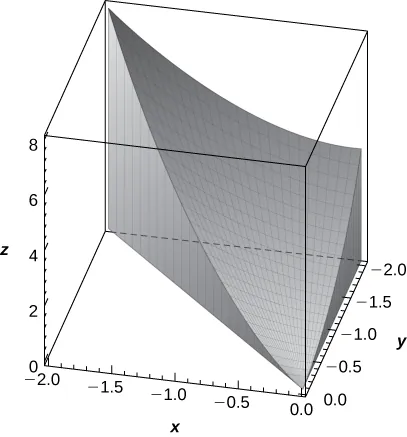215.

$∫01∫13∫24(y2z2+1)dzdxdy;∫01∫13∫24(y2z2+1)dzdxdy;$ $∫01∫13∫24(x2y2+1)dydzdx∫01∫13∫24(x2y2+1)dydzdx$

219.

$V = ∫ − a a ∫ − a 2 − z 2 a 2 − z 2 ∫ x 2 + z 2 a 2 d y d x d z V = ∫ − a a ∫ − a 2 − z 2 a 2 − z 2 ∫ x 2 + z 2 a 2 d y d x d z$

221.

$9 2 9 2$

223.

$156 5 156 5$

225.

a. Answers may vary; b. $12831283$

227.

a. $∫0r∫0r2−x2∫0r2−x2−y2dzdydx;∫0r∫0r2−x2∫0r2−x2−y2dzdydx;$ b. $∫0r∫0r2−y2∫0r2−x2−y2dzdxdy,∫0r∫0r2−y2∫0r2−x2−y2dzdxdy,$ $∫0r∫0r2−z2∫0r2−x2−z2dydxdz,∫0r∫0r2−z2∫0r2−x2−z2dydxdz,$ $∫0r∫0r2−x2∫0r2−x2−z2dydzdx,∫0r∫0r2−x2∫0r2−x2−z2dydzdx,$ $∫0r∫0r2−z2∫0r2−y2−z2dxdydz,∫0r∫0r2−z2∫0r2−y2−z2dxdydz,$ $∫0r∫0r2−y2∫0r2−y2−z2dxdzdy∫0r∫0r2−y2∫0r2−y2−z2dxdzdy$

229.

$3 3$

231.

$250 3 250 3$

233.

$5 16 ≈ 0.313 5 16 ≈ 0.313$

235.

$35 2 35 2$

### Section 5.5 Exercises

241.

$9 π 8 9 π 8$

243.

$1 8 1 8$

245.

$π e 2 6 π e 2 6$

249.

a. $E={(r,θ,z)|0≤θ≤π,0≤r≤4sinθ,0≤z≤16−r2};E={(r,θ,z)|0≤θ≤π,0≤r≤4sinθ,0≤z≤16−r2};$ b. $∫0π∫04sinθ∫016−r2f(r,θ,z)rdzdrdθ∫0π∫04sinθ∫016−r2f(r,θ,z)rdzdrdθ$

251.

a. $E={(r,θ,z)|0≤θ≤π2,0≤r≤3,9−r2≤z≤20−r(cosθ+sinθ)};E={(r,θ,z)|0≤θ≤π2,0≤r≤3,9−r2≤z≤20−r(cosθ+sinθ)};$ b. $∫0π/2∫03∫9−r220−r(cosθ+sinθ)f(r,θ,z)rdzdrdθ∫0π/2∫03∫9−r220−r(cosθ+sinθ)f(r,θ,z)rdzdrdθ$

253.

a. $E={(r,θ,z)|0≤r≤3,0≤θ≤π2,0≤z≤rcosθ+3},E={(r,θ,z)|0≤r≤3,0≤θ≤π2,0≤z≤rcosθ+3},$ $f(r,θ,z)=1rcosθ+3;f(r,θ,z)=1rcosθ+3;$ b. $∫03∫0π/2∫0rcosθ+3rrcosθ+3dzdθdr=9π4∫03∫0π/2∫0rcosθ+3rrcosθ+3dzdθdr=9π4$

255.

a. $y=rcosθ,z=rsinθ,x=z,y=rcosθ,z=rsinθ,x=z,$ $E={(r,θ,z)|1≤r≤3,0≤θ≤2π,0≤z≤1−r2},f(r,θ,z)=z;E={(r,θ,z)|1≤r≤3,0≤θ≤2π,0≤z≤1−r2},f(r,θ,z)=z;$ b. $∫13∫02π∫01−r2zrdzdθdr=256π3∫13∫02π∫01−r2zrdzdθdr=256π3$

257.

$π π$

259.

$π 3 π 3$

261.

$π 4 π 4$

263.

$2 π 3 2 π 3$

265.

$V=π12≈0.2618V=π12≈0.2618$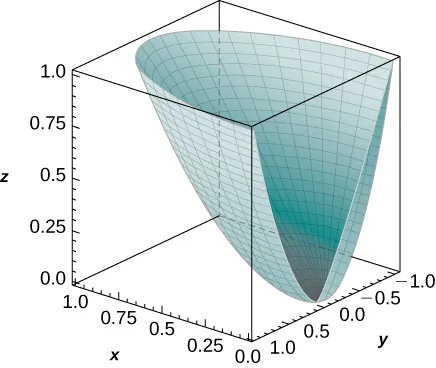267.

$∫ 0 1 ∫ 0 π ∫ r 2 r z r 2 cos θ d z d θ d r ∫ 0 1 ∫ 0 π ∫ r 2 r z r 2 cos θ d z d θ d r$

269.

$180 π 10 180 π 10$

271.

$81 π ( π − 2 ) 16 81 π ( π − 2 ) 16$

277.

a. $f(ρ,θ,φ)=ρsinφ(cosθ+sinθ),f(ρ,θ,φ)=ρsinφ(cosθ+sinθ),$ $E={(ρ,θ,φ)|1≤ρ≤2,0≤θ≤π,0≤φ≤π2};E={(ρ,θ,φ)|1≤ρ≤2,0≤θ≤π,0≤φ≤π2};$ b. $∫0π∫0π/2∫12ρ3cosφsinφdρdφdθ=15π8∫0π∫0π/2∫12ρ3cosφsinφdρdφdθ=15π8$

279.

a. $f(ρ,θ,φ)=ρcosφ;f(ρ,θ,φ)=ρcosφ;$ $E={(ρ,θ,φ)|0≤ρ≤2cosφ,0≤θ≤π2,0≤φ≤π4};E={(ρ,θ,φ)|0≤ρ≤2cosφ,0≤θ≤π2,0≤φ≤π4};$ b. $∫0π/2∫0π/4∫02cosφρ3sinφcosφdρdφdθ=7π24∫0π/2∫0π/4∫02cosφρ3sinφcosφdρdφdθ=7π24$

281.

$π π$

283.

$9 π ( 2 − 1 ) 9 π ( 2 − 1 )$

285.

$∫ 0 π / 2 ∫ 0 π ∫ 0 4 ρ 6 sin φ d ρ d φ d θ ∫ 0 π / 2 ∫ 0 π ∫ 0 4 ρ 6 sin φ d ρ d φ d θ$

287.

$V=4π33≈7.255V=4π33≈7.255$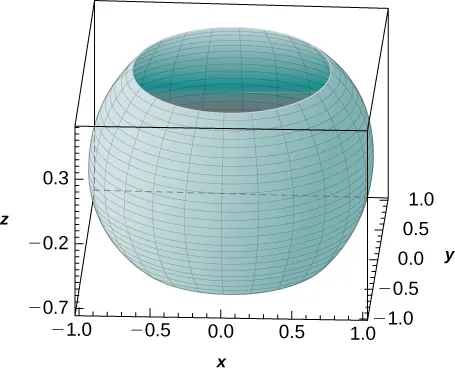289.

$343 π 32 343 π 32$

291.

$∫02π∫24∫−16−r216−r2rdzdrdθ;∫02π∫24∫−16−r216−r2rdzdrdθ;$ $∫π/65π/6∫02π∫2cscφ4ρ2sinρdρdθdφ∫π/65π/6∫02π∫2cscφ4ρ2sinρdρdθdφ$

293.

$P=32P0π3P=32P0π3$ watts

295.

$Q = k r 4 π μ C Q = k r 4 π μ C$

### Section 5.6 Exercises

297.

$27 2 27 2$

299.

$24 2 24 2$

301.

$76 76$

303.

$8 π 8 π$

305.

$π 2 π 2$

307.

$2 2$

309.

a. $Mx=815,My=1625;Mx=815,My=1625;$ b. $x−=125,y−=65;x−=125,y−=65;$
c.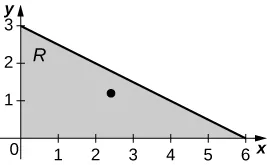311.

a. $Mx=21625,My=43225;Mx=21625,My=43225;$ b. $x−=185,y−=95;x−=185,y−=95;$
c.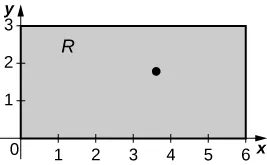313.

a. $Mx=3685,My=15525;Mx=3685,My=15525;$ b. $x−=38895,y−=9295;x−=38895,y−=9295;$
c.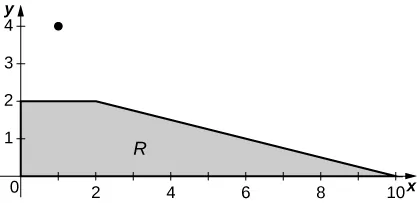315.

a. $Mx=16π,My=8π;Mx=16π,My=8π;$ b. $x−=1,y−=2;x−=1,y−=2;$
c.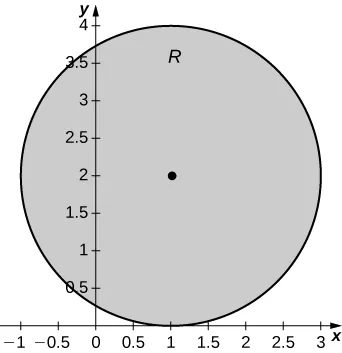317.

a. $Mx=0,My=0;Mx=0,My=0;$ b. $x−=0,y−=0;x−=0,y−=0;$
c.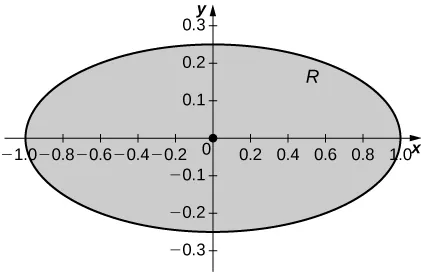319.

a. $Mx=2,My=0;Mx=2,My=0;$ b. $x−=0,y−=1;x−=0,y−=1;$
c.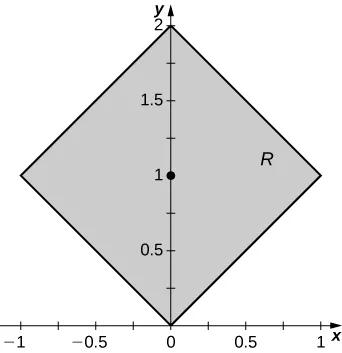321.

a. $Ix=24310,Iy=4865,andI0=2432;Ix=24310,Iy=4865,andI0=2432;$ b. $Rx=355,Ry=655,andR0=3Rx=355,Ry=655,andR0=3$

323.

a. $Ix=259227,Iy=64827,andI0=324027;Ix=259227,Iy=64827,andI0=324027;$ b. $Rx=6217,Ry=3217,andR0=31057Rx=6217,Ry=3217,andR0=31057$

325.

a. $Ix=88,Iy=1560,andI0=1648;Ix=88,Iy=1560,andI0=1648;$ b. $Rx=41819,Ry=741019,Rx=41819,Ry=741019,$ and $R0=2195719R0=2195719$

327.

a. $Ix=128π3,Iy=56π3,andI0=184π3;Ix=128π3,Iy=56π3,andI0=184π3;$ b. $Rx=433,Ry=213,Rx=433,Ry=213,$ and $R0=693R0=693$

329.

a. $Ix=π32,Iy=π8,andI0=5π32;Ix=π32,Iy=π8,andI0=5π32;$ b. $Rx=14,Ry=12,andR0=54Rx=14,Ry=12,andR0=54$

331.

a. $Ix=73,Iy=13,andI0=83;Ix=73,Iy=13,andI0=83;$ b. $Rx=426,Ry=66,andR0=233Rx=426,Ry=66,andR0=233$

333.

$m = 1 3 m = 1 3$

337.

a. $m=9π4;m=9π4;$ b. $Mxy=3π2,Mxz=818,Myz=818;Mxy=3π2,Mxz=818,Myz=818;$ c. $x−=92π,y−=92π,z−=23;x−=92π,y−=92π,z−=23;$ d. the solid $QQ$ and its center of mass are shown in the following figure.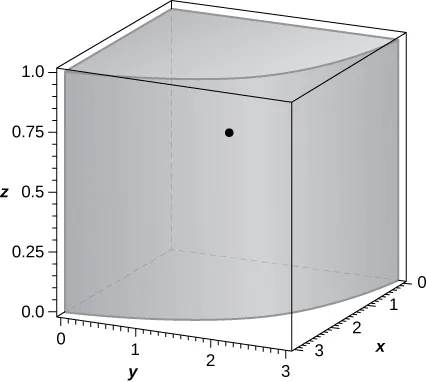339.

a. $x−=322π,y−=3(2−2)2π,z−=0;x−=322π,y−=3(2−2)2π,z−=0;$ b. the solid $QQ$ and its center of mass are shown in the following figure.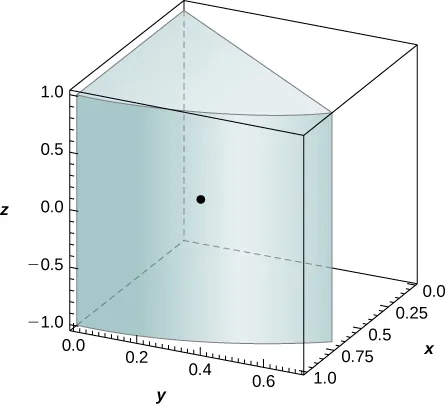343.

$n = −2 n = −2$

349.

a. $ρ(x,y,z)=x2+y2;ρ(x,y,z)=x2+y2;$ b. $16π716π7$

351.

$M x y = π ( f ( 0 ) − f ( a ) + a f ′ ( a ) ) M x y = π ( f ( 0 ) − f ( a ) + a f ′ ( a ) )$

355.

$I x = I y = I z ≃ 0.84 I x = I y = I z ≃ 0.84$

### Section 5.7 Exercises

357.

a. $T(u,v)=(g(u,v),h(u,v)),x=g(u,v)=u2T(u,v)=(g(u,v),h(u,v)),x=g(u,v)=u2$ and $y=h(u,v)=v3.y=h(u,v)=v3.$ The functions $gg$ and $hh$ are continuous and differentiable, and the partial derivatives $gu(u,v)=12,gu(u,v)=12,$ $gv(u,v)=0,hu(u,v)=0andhv(u,v)=13gv(u,v)=0,hu(u,v)=0andhv(u,v)=13$ are continuous on $S;S;$ b. $T(0,0)=(0,0),T(0,0)=(0,0),$ $T(1,0)=(12,0),T(0,1)=(0,13),T(1,0)=(12,0),T(0,1)=(0,13),$ and $T(1,1)=(12,13);T(1,1)=(12,13);$ c. $RR$ is the rectangle of vertices $(0,0),(12,0),(12,13),and(0,13)(0,0),(12,0),(12,13),and(0,13)$ in the $xy-plane;xy-plane;$ the following figure.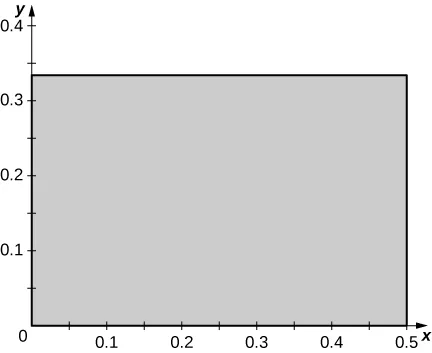359.

a. $T(u,v)=(g(u,v),h(u,v)),x=g(u,v)=2u−v,T(u,v)=(g(u,v),h(u,v)),x=g(u,v)=2u−v,$ and $y=h(u,v)=u+2v.y=h(u,v)=u+2v.$ The functions $gg$ and $hh$ are continuous and differentiable, and the partial derivatives $gu(u,v)=2,gu(u,v)=2,$ $gv(u,v)=−1,gv(u,v)=−1,$ $hu(u,v)=1,hu(u,v)=1,$ and $hv(u,v)=2hv(u,v)=2$ are continuous on $S;S;$ b. $T(0,0)=(0,0),T(0,0)=(0,0),$ $T(1,0)=(2,1),T(1,0)=(2,1),$ $T(0,1)=(−1,2),T(0,1)=(−1,2),$ and $T(1,1)=(1,3);T(1,1)=(1,3);$ c. $RR$ is the parallelogram of vertices $(0,0),(2,1),(1,3),and(−1,2)(0,0),(2,1),(1,3),and(−1,2)$ in the $xy-plane;xy-plane;$ see the following figure.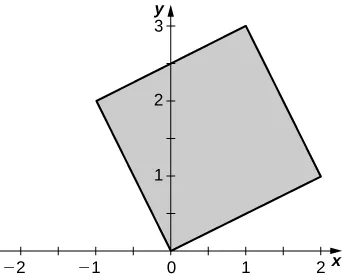361.

a. $T(u,v)=(g(u,v),h(u,v)),x=g(u,v)=u3,T(u,v)=(g(u,v),h(u,v)),x=g(u,v)=u3,$ and $y=h(u,v)=v3.y=h(u,v)=v3.$ The functions $gg$ and $hh$ are continuous and differentiable, and the partial derivatives $gu(u,v)=3u2,gu(u,v)=3u2,$ $gv(u,v)=0,gv(u,v)=0,$ $hu(u,v)=0,hu(u,v)=0,$ and $hv(u,v)=3v2hv(u,v)=3v2$ are continuous on $S;S;$ b. $T(0,0)=(0,0),T(0,0)=(0,0),$ $T(1,0)=(1,0),T(1,0)=(1,0),$ $T(0,1)=(0,1),T(0,1)=(0,1),$ and $T(1,1)=(1,1);T(1,1)=(1,1);$ c. $RR$ is the unit square in the $xy-plane;xy-plane;$ see the figure in the answer to the previous exercise.

363.

$TT$ is not one-to-one: two points of $SS$ have the same image. Indeed, $T(−2,0)=T(2,0)=(16,4).T(−2,0)=T(2,0)=(16,4).$

365.

$TT$ is one-to-one: We argue by contradiction. $T(u1,v1)=T(u2,v2)T(u1,v1)=T(u2,v2)$ implies $2u1−v1=2u2−v22u1−v1=2u2−v2$ and $u1=u2.u1=u2.$ Thus, $u1=u2u1=u2$ and $v1=v2.v1=v2.$

367.

$TT$ is not one-to-one: $T(1,v,w)=(−1,v,w)T(1,v,w)=(−1,v,w)$

369.

$u = x − 2 y 3 , v = x + y 3 u = x − 2 y 3 , v = x + y 3$

371.

$u = e x , v = e − x + y u = e x , v = e − x + y$

373.

$u = x − y + z 2 , v = x + y − z 2 , w = − x + y + z 2 u = x − y + z 2 , v = x + y − z 2 , w = − x + y + z 2$

375.

$S = { ( u , v ) | u 2 + v 2 ≤ 1 } S = { ( u , v ) | u 2 + v 2 ≤ 1 }$

377.

$R = { ( u , v , w ) | u 2 − v 2 − w 2 ≤ 1 , w > 0 } R = { ( u , v , w ) | u 2 − v 2 − w 2 ≤ 1 , w > 0 }$

379.

$3 2 3 2$

381.

$−1 −1$

383.

$2 u v 2 u v$

385.

$v u 2 v u 2$

387.

$2 2$

389.

a. $T(u,v)=(2u+v,3v);T(u,v)=(2u+v,3v);$ b. The area of $RR$ is
$A(R)=∫03∫y/3(6−y)/3dxdy=∫01∫01−u|∂(x,y)∂(u,v)|dvdu=∫01∫01−u6dvdu=3.A(R)=∫03∫y/3(6−y)/3dxdy=∫01∫01−u|∂(x,y)∂(u,v)|dvdu=∫01∫01−u6dvdu=3.$

391.

$− 1 4 − 1 4$

393.

$−1 + cos 2 −1 + cos 2$

395.

$π 15 π 15$

397.

$31 5 31 5$

399.

$T(r,θ,z)=(rcosθ,rsinθ,z);S=[0,3]×[0,π2]×[0,1]T(r,θ,z)=(rcosθ,rsinθ,z);S=[0,3]×[0,π2]×[0,1]$ in the $rθz-spacerθz-space$

403.

The area of $RR$ is $10−46;10−46;$ the boundary curves of $RR$ are graphed in the following figure.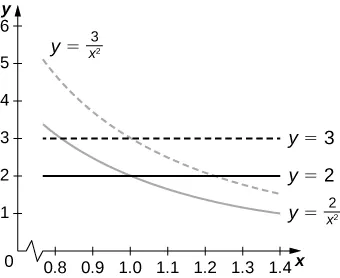405.

$8 8$

409.

a. $R={(x,y)|y2+x2−2y−4x+1≤0};R={(x,y)|y2+x2−2y−4x+1≤0};$ b. $RR$ is graphed in the following figure;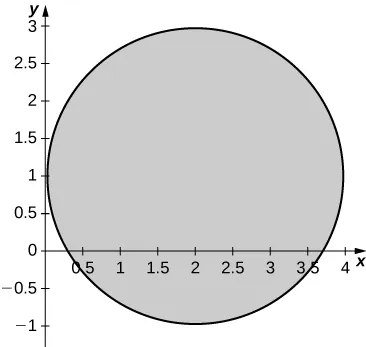c. $3.163.16$

411.

a. $T0,2∘T3,0(u,v)=(u+3v,2u+7v);T0,2∘T3,0(u,v)=(u+3v,2u+7v);$ b. The image $SS$ is the quadrilateral of vertices $(0,0),(3,7),(2,4),and(4,9);(0,0),(3,7),(2,4),and(4,9);$ c. $SS$ is graphed in the following figure;d. $3232$

413.

$2662 3 π ≃ 282.45 in 3 2662 3 π ≃ 282.45 in 3$

415.

$A ( R ) ≃ 83,999.2 A ( R ) ≃ 83,999.2$

### Review Exercises

417.

True.

419.

False.

421.

0

423.

$1 4 1 4$

425.

1.475

427.

$52 3 π 52 3 π$

429.

$π 16 π 16$

431.

93.291

433.

$( 8 15 , 8 15 ) ( 8 15 , 8 15 )$

435.

$( 0 , 0 , 8 5 ) ( 0 , 0 , 8 5 )$

437.

$1.452π×10151.452π×1015$ ft-lb

439.

$y=−1.238×10−7x3+0.001196x2−3.666x+7208;y=−1.238×10−7x3+0.001196x2−3.666x+7208;$ average temperature approximately $2800°C2800°C$

441.

$π 3 π 3$

Order a print copy

As an Amazon Associate we earn from qualifying purchases.